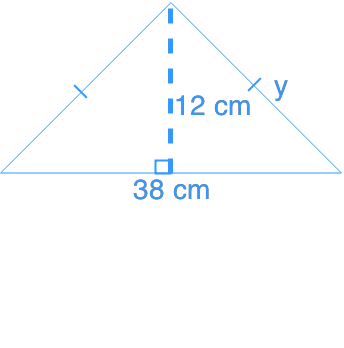Using the pythagorean relationship

Using the pythagorean relationship

In the previous section, we have learned the Pythagorean Theorem. We will now apply the knowledge to answer questions involving compound polygons.

Lessons

• Introduction
a)

i) What is Pythagorean Theorem?

ii) How to use Pythagorean Theorem to find sides of a triangle?

b)
What are Pythagorean Triples?

• 1.